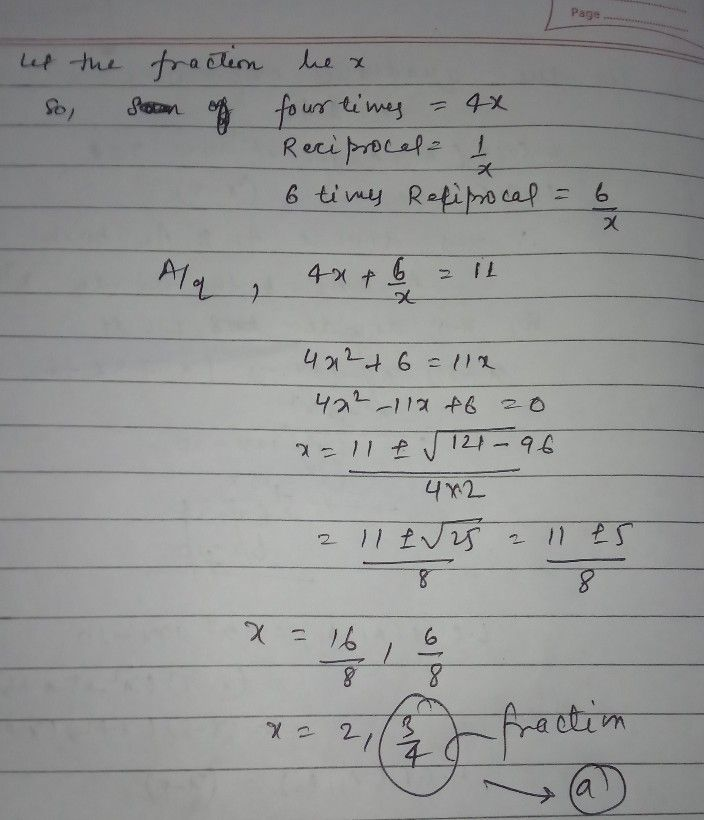Symbol
Problem4. Sum of four times a fraction and $6$ times its reciprocal is $11$ What is the fraction? (a) $314$ (b) $413$ $\left($ $\tarc{O}$ $4/7$ $\left($ (d) $714$ $5$ $A$ fraction is greater than its
10th-13th grade
Other
Question content
sum of four times
SolutionQanda teacher - AMANARUN